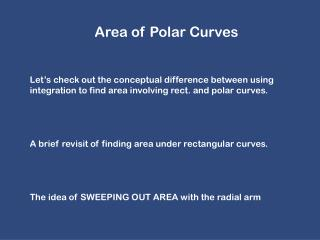DownloadDownload PresentationArea of Polar Curves

# Area of Polar Curves

Download Presentation## Area of Polar Curves

- - - - - - - - - - - - - - - - - - - - - - - - - - - E N D - - - - - - - - - - - - - - - - - - - - - - - - - - -
##### Presentation Transcript

1. Area of Polar Curves • Let’s check out the conceptual difference between using integration to find area involving rect. and polar curves. • A brief revisit of finding area under rectangular curves. • The idea of SWEEPING OUT AREA with the radial arm

2. Let’s check out a section of a polar curve to understand what shape we are using to find area! Now from geometry and our knowledge of circles:

3. Now take the limit as approaches a tiny amount. So small that it will be considered an itty bitty change called Add up a bunch of these super small sectors (physically sweeping the radial arm) from a beginning radial line to an ending radial line. MUST GO COUNTER CLOCKWISE!!!

4. Formally:

5. Let’s get into some awesome examples!!! TOUGH PART IS SELECTING CORRECT LIMITS. GENERAL RULES FOR PICKING LIMITS 1.) Sketch the curve(s) that we will be finding area inside of. (This is where your thorough knowledge of polar curves comes up huge!!!) 2.) “Over what interval must cover so the radial arm sweeps out the region we are finding the area of.

6. Find the area of one leaf of 1.) Sketch – use a table with a few values and the knowledge you can glean from the equation. 2.) Determine the bounds 3.) Integrate - Use symmetry where possible!!! Let’s check out our GC’s polar i-gration ability!

7. a.) w/ geometry b.) w/ integration Be careful in picking limits that only sweep the area out once!!! Double counting…not good.

10. Important to finding the area b/n two separate curves… FINDING POINTS OF INTERSECTION!!! Write points in polar and rectangular form. Graph in your GC. How many points of intersection?

11. 1.) .) Graph to see how many intersections. 2.) Solve by setting the equations equal to each other. This locates the “collisions” – same place and time. 3.) Check the pole (r = 0) for any “non-collisions”. Why the pole? 4.) If not at the pole, then use your graph to find the “non-collisions”

12. Find the points of intersection of the following curves. Rectangular and Polar form

13. Finding Area Between Two Curves 1.) Sketch the graph. 2.) Find the points of intersection. 3.) Use the graph and the intersections to visually figure out the bounds of integration and the integrand. (Don’t be afraid to talk it out like “Cut out the inner loop from this region to get the area”) 4.) Integrate

14. Polar Arc Length From p-metric eqns. we have: We also know we can write polar eqns. in p-metric form:

15. So polar arc length is: Let’s substitute in for each derivative and then simplify to find an awesome formula for polar arc length! MAKE SURE TO ONLY TRACE THE CURVE ONCE!!!

16. SKETCH TO ENSURE ONLY ONE TRACE ON GIVEN INTERVAL

17. Finding Surface Area of a Solid of Revolution Just like Parametric!! Which equation is for rotation about the polar axis? How do you know conceptually?